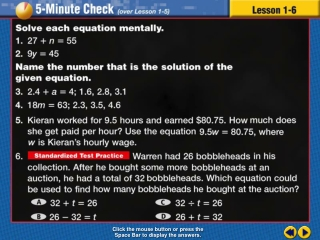# Transparency 6 - PowerPoint PPT PresentationDownload PresentationTransparency 6

Transparency 6
Download Presentation## Transparency 6

- - - - - - - - - - - - - - - - - - - - - - - - - - - E N D - - - - - - - - - - - - - - - - - - - - - - - - - - -
##### Presentation Transcript

1. Transparency 6 Click the mouse button or press the Space Bar to display the answers.

2. Splash Screen

3. Example 6-1b Objective Use addition and multiplication properties to solve problems

4. Example 6-1b Vocabulary Equivalent expressions Expressions that have the same value 4 + 3 = 7 5 + 2 = 7 6 + 1 = 7

5. Example 6-1b Vocabulary Properties Statements that are true for any number or variable

6. Distributive Property 3 (4 + 6) = 3(4) + 3(6)

7. Properties

8. Lesson 6 Contents Example 1Use the Distributive Property Example 2Use the Distributive Property Example 3Use the Distributive Property Example 4Identify Properties Example 5Identify Properties Example 6Identify Properties Example 7Identify Properties

9. Use the Distributive Property to write as an equivalent expression. Then evaluate the expression. Example 6-1a Write the expression 8 ( 5 + 7 ) Distribute the factor 8 to the numbers inside the parentheses 8 ( 5 ) 8 ( 5 ) + 8 ( 5 ) + 8 ( 7 ) Distribute the 8 to the 5 Bring down the + Distribute the 8 to the 7 1/7

10. Use the Distributive Property to write as an equivalent expression. Then evaluate the expression. Example 6-1a Follow the order of operations P E MD AS 8 ( 5 ) + 8 ( 7 ) Multiply first 40 + 56 Multiply 8(5) 96 Bring down + Multiply 8(7) Add 40 + 56 Answer: 96 1/7

11. Use the Distributive Property to write as an equivalent expression. Then evaluate the expression. Example 6-1b Answer: 36 1/7

12. Use the Distributive Property to write as an equivalent expression. Then evaluate the expression. Example 6-2a Write the expression ( 2 + 9 ) 6 Distribute the factor 6 to the numbers inside the parentheses (2)6 (2)6 + (2)6 + (9)6 Distribute the 6 to the 2 Bring down the + Distribute the 6 to the 9 2/7

13. Use the Distributive Property to write as an equivalent expression. Then evaluate the expression. Example 6-2a Follow the order of operations (2)6 + (9)6 P E MD AS Multiply first 12 + 54 Multiply (2)6 66 Bring down + Multiply (9)6 Add 12 + 54 Answer: 66 2/7

14. Use the Distributive Property to write as an equivalent expression. Then evaluate the expression. Example 6-2b Answer: 56 2/7

15. Example 6-3a VACATIONSMr. Harmon has budgeted \$150 per day for his hotel and meals during his vacation. If he plans to spend six days on vacation, how much willhe spend? Decide how to solve the problem 6 (150) Spend \$150 per day 6 days Remember: per refers to multiplication Write expression 3/7

16. Example 6-3a VACATIONSMr. Harmon has budgeted \$150 per day for his hotel and meals during his vacation. If he plans to spend six days on vacation, how much willhe spend? Use the distributive property to solve 6 (150) Decompose 150 6 (100 + 50) Distribute the factor 6 to the numbers inside the parentheses 6(100) 6(100) + 6(100) + 6(50) Distribute the 6 to the 100 Bring down the + Distribute the 6 to the 50 3/7

17. Example 6-3b Follow the order of operations P E MD AS 6(100) + 6(50) Multiply first Multiply 6(100) + 600 300 Bring down + 900 Multiply 6(50) Add 600 + 300 Label answer Answer: \$900 spent on trip 3/7

18. Example 6-3c COOKIESHeidi sold cookies for \$2.50 per box for a fundraiser. If she sold 60 boxes of cookies, how much money did she raise? Answer: \$150 3/7

19. Name the property shown by the statement Example 6-4a  7 = 1  7 Write problem Determine property Identity Property Multiplying by 1 keeps the same identity of the number Of Multiplication The process is multiplying Answer: Identity Property of Multiplication 4/7

20. Name the property shown by the statement . Example 6-4b  Answer: Identity Property of Multiplication 4/7

21. Name the property shown by the statement Example 6-5a 24 + 5 = 5 + 24 Write problem Determine property Commutative Property The numbers have been switched with each other but everything else remains the same Of Addition Refers to the Commutative Property The process is adding Answer: Commutative Property of Addition 5/7

22. Name the property shown by the statement Example 6-5b Answer: Commutative Property of Addition 5/7

23. Name the property shown by the statement Example 6-6a 7 + 0 = 7 Write problem Determine property Identity Property Adding by 0 keeps the same identity of the number Of Addition The process is adding Answer: Identity Property of Addition 6/7

24. Name the property shown by the statement Example 6-6b Answer: Identity Property of Addition 6/7

25. Name the property shown by the statement Example 6-7a     (11  4)  8 = 11  (4  8) Write problem Determine property Associative Property Numbers in same order but have been regrouped with parenthesis Of Multiplication The process is multiplying Answer: Associative Property of Multiplication 7/7

26. Name the property shown by the statement Example 6-7b * Answer: AssociativeProperty of Addition 7/7

27. End of Lesson 6 Assignment